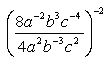What is the answer to the following problem: (8a to the negative 2 b cube c to the negative 4/4a squared b to the negative 3 c squared) to the negative 2 Hi there. I think your description matches this expression:When you multiply terms that have the same base, you add their exponents. When you divide such terms, you subtract their exponents. For example, a2 divided by a2 equals a2-2. Use this rule to simplify what is inside the parentheses for all your terms. When you have a term with an exponent, such as a-4, and you raise that to another exponent, you multiply the exponents. For example, (a-4)-2 equals a(-2)(-4), which is a8. Use this rule to apply the exponent outside the parentheses to the exponents inside the parentheses. Remember to apply it to the numbers inside the parentheses as well as the variables. Hope this helps, Stephen La Rocque.